# HBSE 6th Class Maths Solutions Chapter 7 Fractions Ex 7.3

Haryana State Board HBSE 6th Class Maths Solutions Chapter 7 Fractions Ex 7.3 Textbook Exercise Questions and Answers.

## Haryana Board 6th Class Maths Solutions Chapter 7 Fractions Exercise 7.3

Question 1.
Write the fractions. Are all these fractions equivalent ?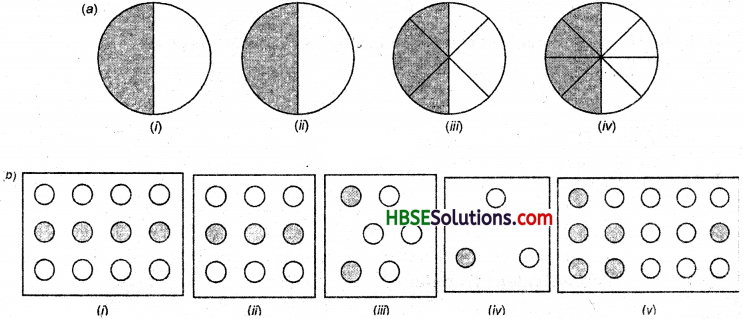Solution:
(a) $$\frac{1}{2}, \frac{2}{4}, \frac{3}{6}, \frac{4}{8}$$. All these fractions are equivalent.
(b) ,$$\frac{4}{12}, \frac{3}{9}, \frac{2}{6}, \frac{1}{3}, \frac{6}{15}$$. First four fractions are equivalent. All fractions are not equivalent. 14 a bo 15

Question 2.
Write the fractions and pair up the equivalent fraction from each row A and B.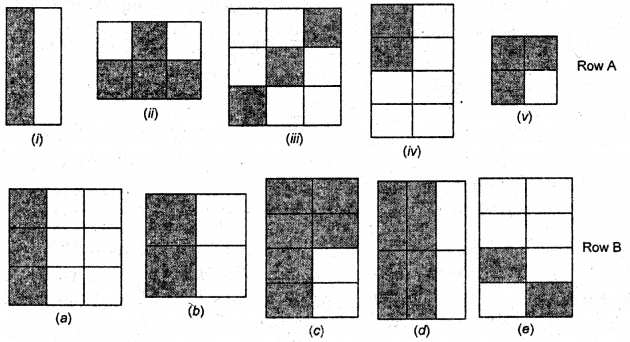Solution: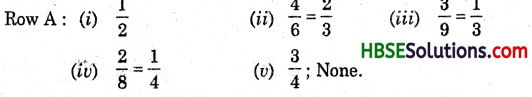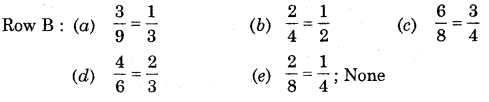Question 3.
Replace in each of the following by the correct number: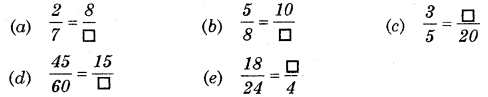Solution: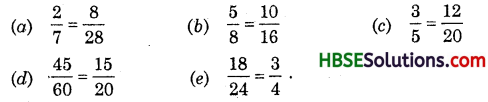Question 4.
Find the equivalent fraction of $$\frac{3}{5}$$ having
(a) denominator 20
(b) numarator 9
(c) denominator 30
(d) numarator 27
Solution: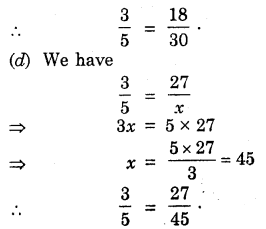Question 5.
Find the equivalent fraction of $$\frac{36}{48}$$ with
(a) numarator 9
(b) denominator 4
Solution: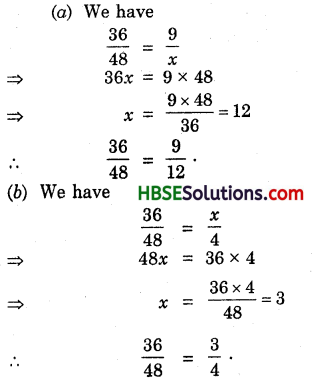Question 6.
Check whether the given fractions are equivalent :
(a) $$\frac{5}{9}, \frac{30}{54}$$
(b) $$\frac{3}{10}, \frac{12}{50}$$
(c) $$\frac{7}{13}, \frac{5}{11}$$
Solution: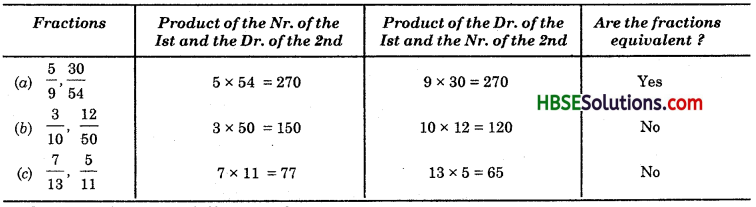Question 7.
Reduce the following fractions to their simplest forms :
(a) $$\frac{48}{60}$$
(b) $$\frac{150}{60}$$
(c) $$\frac{84}{98}$$
(d) $$\frac{12}{52}$$
(e) $$\frac{7}{28}$$
Solution: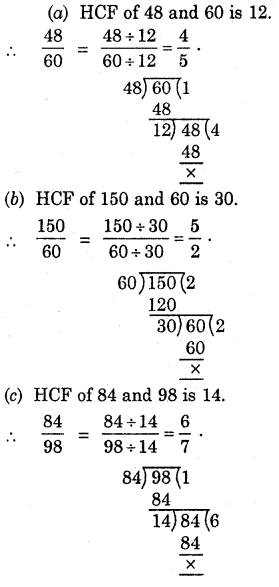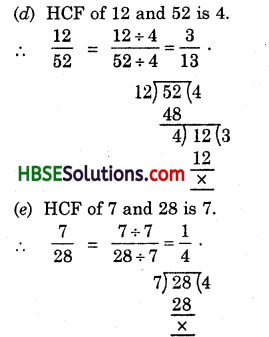Question 8.
Ramesh had 20 pencils, Sheelu had 50 pencils and Jamaal had 80 pencils. After 4 months, Ramesh used up 10 pencils, Sheelu used up 25 pencils, and Jamaal used up 40 pencils. What fraction did each use up ? Check if each has used up an equal fraction of their pencils ?
Solution:
∵ Ramesh had 20 pencils and he used up 10 pencils.
Fraction of pencils used by Ramesh = $$\frac{10}{20}=\frac{1}{2}$$
∵ Sheelu had 50 pencils and she used up 25 pencils.
∴ Fraction of pencils used by Sheelu = $$\frac{25}{50}=\frac{1}{2}$$
∵ Jamaal had 80 pencils and he used up 40 pencils.
∴ Fraction of pencils used up by Jamaal = $$\frac{40}{80}=\frac{1}{2}$$
Yes, each had used up an equal fraction of their pencils.

Question 9.
Match the equivalent fractions and write other 2 for each :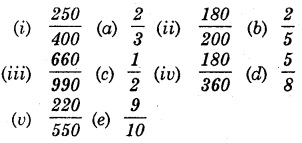Solution:
Solution:
To reduce $$\frac{250}{400}$$ we find the H .C.F. of 250 and 400.
We have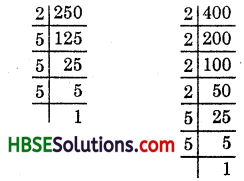∴ 250 = 2 x 5 x 5 x 5
and 400 = 2 x 2 x 2 x 2 x 5 x 5
∴ H.C.F. of 250 and 400
= 2 x 5 x 5 = 50
$$\frac{250}{400}=\frac{250 \div 50}{400 \div 50}=\frac{5}{8}$$
Thus (i) is matched to (d).

(ii) To reduce $$\frac{180}{200}$$ we find the HCF of 180 and 200.
We have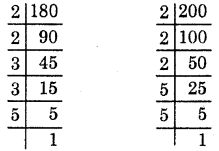∴ 180 = 2 x 2 x 3 x 3 x 5
and 200 = 2 x 2 x 2 x 5 x 5
∴ HCF of 180 and 200
= 2 x 2 x 5 = 20
$$\frac{180}{200}=\frac{180 \div 20}{200 \div 20}=\frac{9}{10}$$
Thus, (ii) is matched to (e).(iii) To reduce $$\frac{660}{990}$$, we find the HCF of
660 and 990.
We have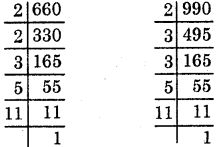∴ 660 = 2 x 2 x 3 x 5 x 11
and 990 = 2 x 3 x 3 x 5 x 11
∴ HCF of 660 and 990
= 2 x 3 x 5 x 11 = 330
$$\frac{660}{990}=\frac{660 \div 330}{990 \div 330}=\frac{2}{3}$$
Thus, (iii) is matched to (a).

(iv) To reduce $$\frac{180}{360}$$, we find the HCF of 180 and 360.
∴ 180 = 180
360 = 180 x 2
∴ HCF of 180 and 360 = 180

Thus, (iv) is matched to (c).(v) To reduce $$\frac{220}{550}$$, we find the HCF of 220
and 550 We have
220 = 550
and 220 = 2 x 110
HCF of 220 and 550 = 110

Thus, (v) is matched to (b).